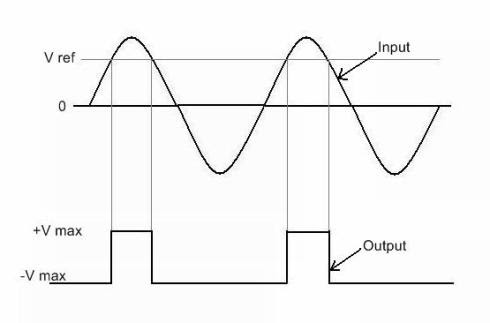# Op-Amp comparator - working, waveforms and analysis

## Op-Amp Comparator:

In this section we are going to learn a basic op-amp application as a comparator. Comparator is a circuit used for comparing two voltages (either DC or both AC or one DC & one AC) and indicating the relationship between those voltages.

Generally comparators are used to compare either:

a)      Two changing voltages to each other, example: two different sinusoidal waveforms.

b)      A changing voltage to a set DC reference voltage.

The circuit diagram of op-amp comparator is sown below. There is no feedback path present in the circuit. To understand the working of op-amp comparator let us consider a sinusoidal input voltage is applied to the non-inverting terminal where as a fixed DC voltage (V reference) is applied to the inverting terminal.

### Circuit diagram:

[caption id="attachment_5880" align="aligncenter" width="300"]op amp comparator[/caption]

In this example we are going to compare sinusoidal voltage with fixed dc voltage using op-amp comparator. The working of this comparator is explained as follows.

### Working:

As long as the input voltage is below the reference voltage (which is connected to the non-inverting terminal) , the comparator output is approximately “-Vmax” volts. When input voltage equals to reference voltage or exceeds, the output voltage of the comparator becomes “+Vmax” volts. Thus op-amp comparator shows the relationship between the magnitudes of two voltages applied to its input. Following figure shows the polarity (or magnitude) relationship between two voltages.

[caption id="attachment_5038" align="aligncenter" width="490"]op amp comparator waveforms[/caption]

Now let us discuss one special case of op-amp comparator. If we apply reference voltage (Vref) to the inverting terminal and made it ground so that V ref =0V. Now in this case the output of the comparator will be “- V max” volts as long as the input voltage is below 0V. When input voltage exceeds 0V the output of the comparator switches to “+V max” volts.

Such special case op-amp comparator is called as zero-level detector. This zero-level detector circuit can be used to obtain square waveform from a sinusoidal waveform.